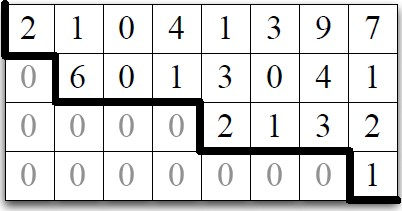# Linear Algebra - Triangular Matrix

$$\begin{bmatrix} 1 & 0 & 2 & 4 \\ 0 & 3 & 3 & 2 \\ 0 & 0 & 1 & 5 \\ 0 & 0 & 0 & 2 \end{bmatrix}$$

The matrix is a triangular matrix. Definition: An n x n upper triangular matrix A is a matrix with the property that $A_(ij) = 0 for j > i$ . The entries forming the triangle can be be zero or nonzero. We can use backward substitution to solve such a matrix-vector equation.

The Echelon matrix is a generalization of triangular matrices.

## Triangular linear systems as a matrix-vector dot product

Triangular linear systems as a matrix-vector dot product $$\begin{array}{l|l} \text{The following triangular linear systems} & \textrm{can be expressed as} \\ \hline \\ \begin{array}{rrrrrrrr} [ & 1, & 0.5, & -2, & 4 & ] & . & v & = & -8 \\ [ & 0, & 3, & 3, & 2 & ] & . & v & = & 3 \\ [ & 0, & 0, & 1, & 5 & ] & . & v & = & -4 \\ [ & 0, & 0, & 0, & 2 & ] & . & v & = & 6 \end{array} & \begin{bmatrix} 1 & 0.5 & -2 & 4 \\ 0 & 3 & 3 & 2 \\ 0 & 0 & 1 & 5 \\ 0 & 0 & 0 & 2 \end{bmatrix} * [-8,3,-4,-6] \end{array}$$

## Computation

Solving a triangular linear system with Backward substitution in Python:

# rowlist is a list of vector Ex.: [{2,3,-4},{1,2},{5}]
# b are the value. Ex: [10,3,15]
# label list define the domain {A,B,C} of {1,2,4}. Ex: [0,1,2]
def triangular_solve(rowlist, label_list, b):
# Initialize vector x to zero vector
x = zero_vec(set(label_list))
for r in reversed(range(len(rowlist))):
c = label_list[r]
# Procedure populate x entry by entry.
x[c] = (b[r] - x*rowlist[r])/rowlist[r][c]
return x


Discover MoreLinear Algebra - (Linear system|System of Linear equations)

In mathematics, a system of linear equations (or linear system) is a collection of linear equations involving the same set of variables. Each linear system corresponds to a linear system with zero...Linear Algebra - Matrix

The Traditional notion of a matrix is: a two-dimensional array a rectangular table of known or unknown numbers One simple role for a matrix: packing together a bunch of columns or rows Matrix...Linear Algebra - Matrix Vector (Multiplication)

There is two ways to multiply a matrix by a vector: matrix vector or vector matrix For each of these multiplication, two equivalent implementations (definitions): in terms of linear combinations...Linear Algebra - Orthogonalization - Building an orthogonal set of generators

Original stated goal: Find the projection of b orthogonal to the space V spanned by arbitrary vectors Input: A list of vectors over the reals output: A list of mutually orthogonal vectors such...Linear System - Echelon Matrix

The Echelon form is a generalization of triangular matrices. An matrix A is in echelon form if it satisfies the following condition: for any row, if that row’s first nonzero entry is in position...